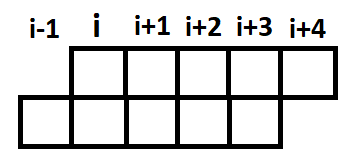# [APIO/CTSC2007]动物园

## 题意

动物园里有n只动物从1到n编号。有c个小朋友，每个小朋友可以看到连续的5只动物(所在的围栏)。其中有他们各自喜欢的和不喜欢的。
现在可以扔掉一些动物(围栏还在)，然后对于1个小朋友，只要看到1只他喜欢的动物还在，或者一只他不喜欢的动物被扔了，他就会高兴...求最多让几个小朋友高兴。

## 补充

似乎有很多人被出题人一句不经意的话误导了...

“你可以选择将一些动物从围栏中移走以使得小朋友不会害怕。但你不能移走所有的动物，否则小朋友们就没有动物可看了。”

在数据中，这句话是假的...也就是说，我们其实是可以把所有动物都扔掉的.

## 分析

特别像网络流...当场被数据范围踩在脚下
发现5非常非常小，考虑状压dp，算起来复杂度很妙的样子~。
我们对于每一个围栏的位置i,显然在i上的小朋友能看到的区间为$\left\{\begin{array}{cc}\left [ i,i+4 \right ], & i\leq n-4\\ \left [ i,i+4-n \right ], & n-4<i \leq n\end{array}\right.$
即[i,(i+4)%n]我们可以把这5个值状压在一起，在每一位上用0/1表示一只动物扔/不扔。
接着能很快得到dp状态：用f[i][j]表示从位置i开始的5个位置状态为j时，从位置1到i的最多的高兴的小朋友的数量
从i-1转移到i，只需讨论i-1位置是否取即可。很容易yy出fake的转移方程：$f[i][j]=max\lbrace f[i-1][j>>1],f[i-1][(j>>1)+16]\rbrace+i点的贡献$将就着看
(j>>1取出了前4位状态，是否加16决定了i-1位是否为1)

### 为什么能加上i点的贡献？(或为什么不加别的点的贡献)(或为什么我这么菜)

因为直到枚举到i时，在位置i的小朋友能看到的5个围栏才都包括在了状态中，固可以只考虑i点，防止答案的遗漏和重复计算。

### 那么问题来了：如何计算i点贡献？

很简单，在读入小朋友喜欢和不喜欢的动物时，直接预处理出g[i][j]表示i位置看到的5只动物的状态为j时，能让多少待在i的小朋友高兴

最后，由于动物园是个环，我们要先枚举一个断点的状态才能往后转移，并且转移一圈回来之后最后的答案也必须取枚举的那个状态。

## 代码

#include <iostream>
#include <cstdio>
#include <cctype>
#define il inline
#define vd void
#define rep(i,x,y) for(register int i=x;i<=y;++i)
#define drp(i,x,y) for(register int i=x;i>=y;--i)
using namespace std;
const int Len=2333333,mn=1e4+5;
char buf[Len],*p1=buf,*p2=buf,duf[Len],*q1=duf;
il char gc(); il int rd(); il vd pc(char c); il vd rt(int x); il vd flush();
template<class T> il T Max(T a,T b){return a>b?a:b;}
int n,c,id,a,b,x,y,ans,f,g[mn],dp;
int main(){
n=rd(),c=rd();
while(c--){
id=rd(),a=rd(),b=rd(),x=y=0;
while(a--) x|=1<<4-(rd()-id+n)%n; //(rd()-id+n)%n为结束点编号，用4去减实现了反转
while(b--) y|=1<<4-(rd()-id+n)%n;
rep(j,0,31) if(~j&x||j&y) ++g[j][id]; //~是优先级高的按位取反
}//我的dp滚了一维，大家自行吐槽
rep(o,0,31){
rep(i,0,31) dp[i]=-2e9;
dp[o]=f=0; //除了状态o以外的状态都为极小值(不可达到)，位置0其实对应位置n
rep(i,1,n){f^=1;
rep(j,0,31) dp[f][j]=Max(dp[f^1][j>>1],dp[f^1][(j>>1)+16])+g[j][i];
}
ans=Max(ans,dp[f][o]); //最后算一圈又算出dp[n][o]
}
rt(ans);
return flush(),0;
}

il vd flush(){fwrite(duf,1,q1-duf,stdout);}